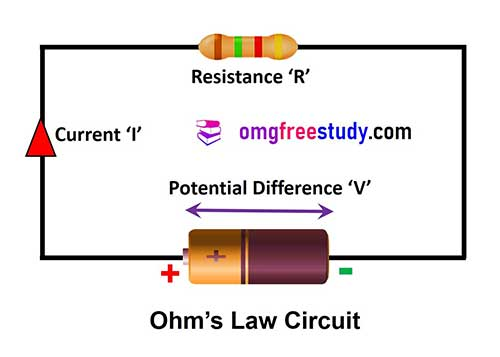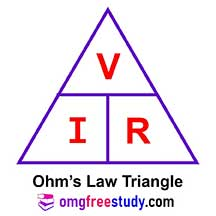# What is Ohm’s Law? | Ohm’s Law Triangle

Before understanding the ohm’s law we need to understand basic concept of electrical circuit.

In the given electric circuit, the current ‘I’ flowing and completes its path from the positive terminal of the battery via Resistance ‘R’ and load back to the negative terminal of the battery.The circuit shown in Figure is a closed path circuit. In order to make any circuit to function normally the following three factors are essential.

1) Electromotive force (EMF) to flow the electrons through the circuit.

2) Current (Ι), the flow of electrons.

3) Resistance (R), the opposition to limit the flow of electrons or current.

## Ohm’s law

George Simon Ohm’s in 1826 discovered that for metallic conductor, there is a significant constant ratio of the potential difference (V) between two ends of the conductor.

Ohm’s law gives the relation between the current, voltage and resistance of a circuit.

Ohm’s law states that the ratio of the voltage (V) across any two points in a circuit to the current (I) flowing through is constant, when physical conditions, namely temperature etc. remain constant. This constant is denoted as resistance (R) of the circuit.

Or

In simple, Ohm’s law states that in any electrical closed circuit, the current (I) is directly proportional to the voltage (V), and it is inversely proportional to the resistance ‘R’ if temperature is constant.

i.e. I proportional to V (When ‘R’ is kept constant),

I proportional to R (When ‘V’ is kept constant),

I proportional to V/R (Relation between I, V and R)

It means that,

I = V/R

Where,

V = Voltage applied to the circuit in ‘Volt’,

I = Current flowing through the circuit in ‘Amp’,

R = Resistance of the circuit in Ohm (Ω),

Written as a mathematical expression for Ohm’s Law is

Resistance (R)= Voltage (V) / Current (I)

## Ohm’s Law Triangle

The above relationship can be represented in a triangle as shown in Figure. In this triangle whatever the value you want to find out, place the finger on it then the remaining position of the other factors will give you the required value.For example for finding ‘V’ close the value ‘V’ then remaining values are IR,

so V = IR.

Again for finding ‘R’, close the value R, then remaining values are V/I,

so R = V/I, in this way we can find one quantity out of three by this quick method.

of Module 4: Basic Electrical Practice

• Ohm’s law – simple electrical circuits and problems.
• Kirchhoff’s law and its applications.
• DC series and parallel circuits.
• Open and short circuit in series and parallel network.
• Laws of resistance and various types of resistors.
• Wheatstone bridge – principle and its application.
• Effect of variation of temperature on resistance.
• Series and parallel combination circuit

MCQ Questions PDF

This MCQ Question Is Taken From Bharat Skill Website Published By NIMI.

Objective questions on the above topics explain in the below video on YouTube.

Scroll to Top
Scroll to Top